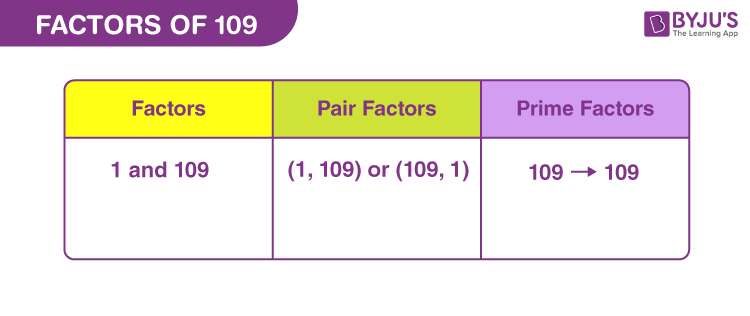# Factors of 109

Factors of 109 are the natural numbers that divide the original number, evenly or uniformly. If factor divides 109, then there should be zero remainder left. Therefore, the factor is also called the divisor of 109. But, 109 is a prime number, therefore, there are only two possible factors.

When two factors are multiplied in a pair, to get the original number, then such factors are called pair factors. Let us find the factors, pair factors and prime factors of 109 in this article.## How to Find the Factors of 109?

Factors of a number divide it into equal parts. Thus, the remainder should be zero.

 109 ÷ 1 = 109 [Remainder = 0] 109 ÷ 109 = 1 [Remainder = 0]

Therefore, there are only two factors of 109. They are 1 and 109.

Let us see if 109 is divisible by any other number.

109 ÷ 2 = 54.5

109 ÷ 5 = 21.8

109 ÷ 10 = 10.9

109 ÷ 58 = 1.87931034483

109 ÷ 100 = 1.09

Likewise, if we divide 109 by any other number, then the result will not be a whole number. Therefore, there are only two possible factors.

## Pair Factors of 109

The pair factors of 109 results in the original number, while we multiply them in a pair.

• 1 × 109 = 109
• 109 × 1 = 109

Therefore, the pair factors of 109 are (1, 109) or (109, 1).

If we multiply two negative integers to get the original number, then we have:

• -1 × -109 = 109
• -109 × -1 = 109

Therefore, the negative pair factors are (-1, -109) or (-109, -1).

## Prime Factorisation of 109

109 is a prime number itself. The prime factorisation of 109 will result in the original only, because it is divisible by itself.

109 ÷ 109 = 1

 Prime factorisation of 109 = 109 Exponential form = 1091

## Solved Examples

Q.1: Find the sum of factors of 109. Also, find the average

Solution: The factors of 109 are 1 and 109.

Sum = 1 + 109 = 110

Thus, 110 is the required sum.

Average = Sum ÷ Total number of factors

Average = 110 ÷ 2 = 55

Therefore, the required average is 55.

Q.2: What are the GCF of 109 and 158?

Solution: The factors of respective numbers are:

109 → 1, 109

158 → 1, 2, 79, 158

Therefore, the GCF of 109 and 158 is 1.

Q.3: What are the common factors of 109 and 218?

Solution: The factors of the respective numbers are:

109 → 1, 109

218 → 1, 2, 109, 218

Therefore, the common factors of 109 and 218 are 1 and 109.

Q.4: What is the GCF of 109 and 271?

Solution: The factors are:

109 → 1, 109

271 → 1, 271

Therefore, the GCF is 1.

### Practice Questions

1. What are the divisors of 109?
2. How many factors does 109 have?
3. What is the GCF of 109 and 111?
4. What are the common factors of 109 and 100?
5. Find if 109 and 108 have any common factors.

## Frequently Asked Questions on Factors of 109

### What are the factors of 109?

The factors of 109 are 1 and 109.

### Is 109 divisible by any other number?

109 is not divisible by any other number apart from 1 and 109.

### What are the multiples of 109?

The multiples of 109 are 109, 218, 327, 436, 545, 654, 763, 872, 981, 1090 and so on.

### Is 109 a prime number or composite number?

109 is a prime number because it has only two factors.

### Is 109 a perfect square number?

No, 109 is not a perfect square because the square root of 109 is not a whole number.